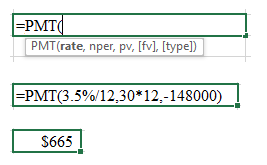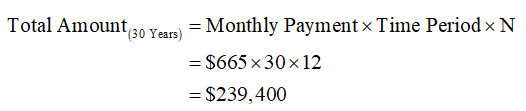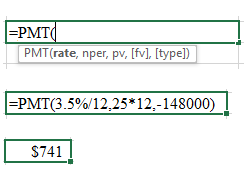# Suppose you want to buy a \$148,000 home. You found a bank that offers a 30-year loan at 3.5% APR.What will be your monthly payment? (Round to the nearest cent.)\$How much would you end up paying the bank for the home after 30 years? (Round to the nearest cent.)\$Suppose you wanted to reduce the time of your loan to 25 years. What would be your new monthly payment? (Round to the nearest cent.)\$How much would you end up paying the bank for the home after 25 years? (Round to the nearest cent.)\$How much did you save by reducing the time of your mortgage loan? (Round to the nearest cent.)\$

Question
3 views
Suppose you want to buy a \$148,000 home. You found a bank that offers a 30-year loan at 3.5% APR.

What will be your monthly payment? (Round to the nearest cent.)

\$

How much would you end up paying the bank for the home after 30 years? (Round to the nearest cent.)

\$

Suppose you wanted to reduce the time of your loan to 25 years. What would be your new monthly payment? (Round to the nearest cent.)

\$

How much would you end up paying the bank for the home after 25 years? (Round to the nearest cent.)

\$

How much did you save by reducing the time of your mortgage loan? (Round to the nearest cent.)

\$

check_circle

Step 1

Loan Amount = \$148,000

Time Period = 30 years

Interest Rate = 3.5%

The calculation of the monthly payment using the PMT function of the excel is as follows:

To open the "PMT function" window - MS-Excel --> Formulas --> Financials --> PMT.Hence, the monthly payment is \$665.

Step 2

Monthly Payment = \$665

Time Period = 30 years

Number of Payments in a Period (N) = 12

The calculation of the total amount paid at the end of 30 years is as follows:Hence, the total amount paid at the end of 30 years is \$239,400.

Step 3

Loan Amount = \$148,000

Time Period = 25 years

Interest Rate = 3.5%

The calculation of the monthly payment using the PMT function of the excel is as follows:

To open the "PMT function" window - MS-Excel --> Formulas --> Financials --> PMT.Hence, the mon...

### Want to see the full answer?

See Solution

#### Want to see this answer and more?

Solutions are written by subject experts who are available 24/7. Questions are typically answered within 1 hour.*

See Solution
*Response times may vary by subject and question.
Tagged in